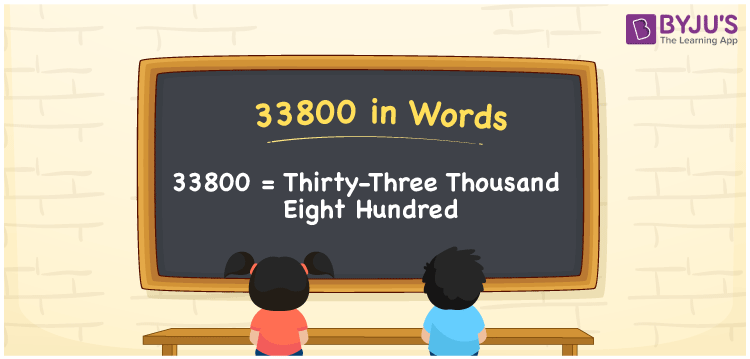# 33800 in Words

33800 in words can be written as Thirty-three Thousand Eight Hundred. The article of 33800 in words will provide students with a clear knowledge of the concept of counting numbers. If Rs. 33800 is spent on buying a new sofa set, then you can say that “I spent Thirty-three Thousand Eight Hundred Rupees in buying a new sofa set”. The  concept of converting 33800 into words using the English alphabet is discussed here. Students will be able to convert numbers in words with the help of the resources available at BYJU’S. 33800 can be read as “Thirty-three Thousand Eight Hundred” in English.

 33800 in words Thirty-three Thousand Eight Hundred Thirty-three Thousand Eight Hundred in Numbers 33800

## 33800 in English Words## How to Write 33800 in Words?

In this section you will learn in detail about conversion of 33800 into words using the place value chart. Five digits are present in 33800. The place value chart of 33800 can be understood easily using the table below.

 Ten Thousands Thousands Hundreds Tens Ones 3 3 8 0 0

The expanded form of the number 33800 is given below:

3 x Ten Thousand + 3 × Thousand + 8 × Hundred + 0 × Ten + 0 × One

= 3 x 10000 + 3 × 1000  + 8 × 100 + 0 × 10 + 0 × 1

= 30000 + 3000 + 800

= 33800

= Thirty-three Thousand Eight Hundred

Hence, 33800 in words is written as Thirty-three Thousand Eight Hundred.

33800 is a natural number that precedes 33801 and succeeds 33799.

33800 in words – Thirty-three Thousand Eight Hundred

Is 33800 an odd number? – No

Is 33800 an even number? – Yes

Is 33800 a perfect square number? – No

Is 33800 a perfect cube number? – No

Is 33800 a prime number? – No

Is 33800 a composite number? – Yes

## Frequently Asked Questions on 33800 in Words

Q1

### Write 33800 in words.

33800 can be written as “Thirty-three Thousand Eight Hundred” in words.
Q2

### Is 33800 an odd or even number?

33800 is an even number because it is divisible by 2.
33800/2 = 16900
Q3

### Write Thirty-three Thousand Eight Hundred in numbers.

Thirty-three Thousand Eight Hundred can be written in numbers as 33800.